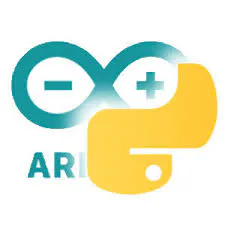# NeoMatrix Arduino Pong

Use an Arduino NeoPixel matrix to create a simple 1 player pong game!

IntermediateFull instructions provided4 hours7,953

## Things used in this project

### Hardware components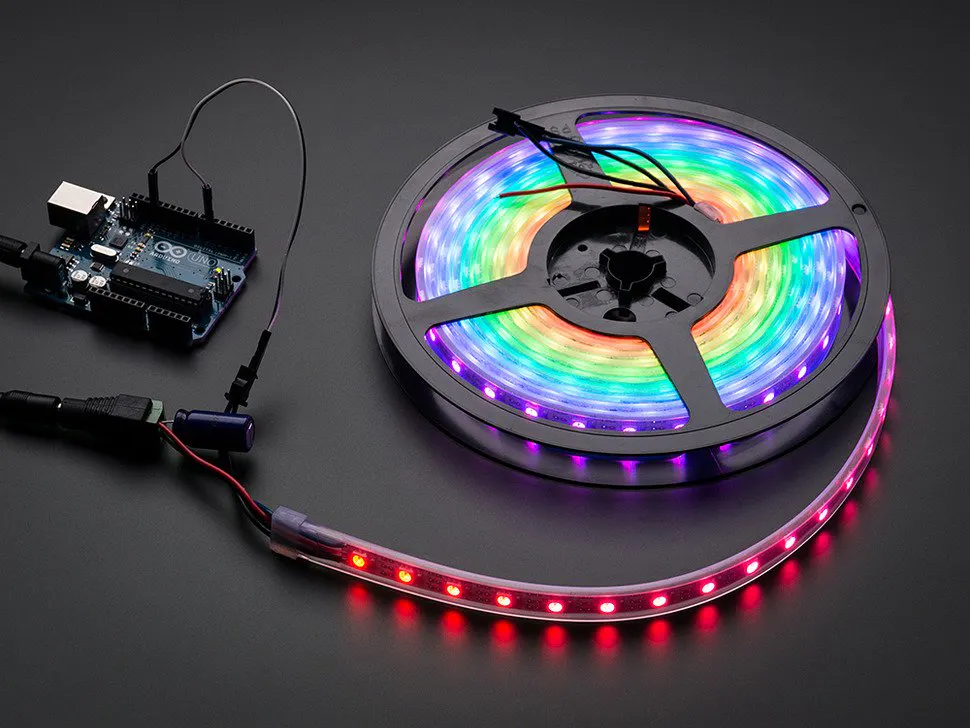NeoPixel strip
×1Jumper wires (generic)
×1Arduino Nano R3
×1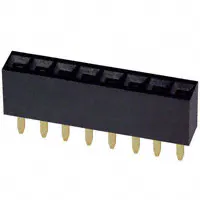Female Header 8 Position 1 Row (0.1")
×1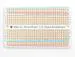×1
 Analog joystick (Generic)
×1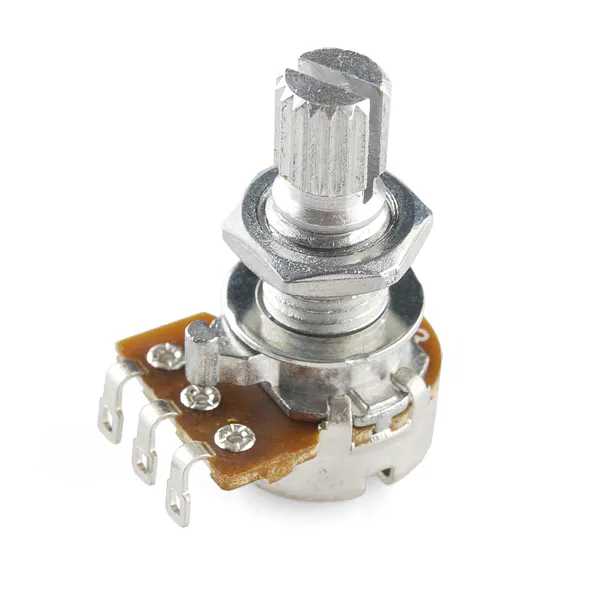Rotary potentiometer (generic)
×1

### Software apps and online servicesArduino IDE

### Hand tools and fabrication machinesSoldering iron (generic)

## Schematics

### Schematic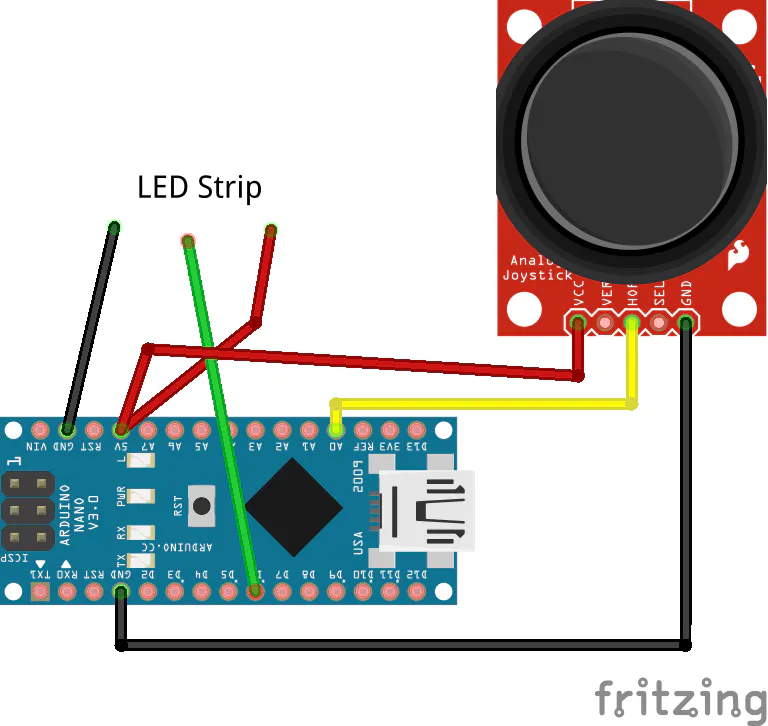## Code

### The Arduino Code

C/C++
```#include <Adafruit_GFX.h>

#define PIN 6

NEO_MATRIX_BOTTOM     + NEO_MATRIX_LEFT +
NEO_MATRIX_COLUMNS + NEO_MATRIX_ZIGZAG,
NEO_GRB            + NEO_KHZ800);

// variables for the position of the ball and paddle
int ballDirectionX = 1;
int ballDirectionY = 1;
int score = 0;

int ballSpeed = 10; // lower numbers are faster

int ballX, ballY, oldBallX, oldBallY;

void setup() {
Serial.begin(9600);
// initialize the display
matrix.begin();
// black background
matrix.fillScreen(0);
matrix.setTextColor(matrix.Color(0,255,0));
matrix.print("GO");
matrix.show();
delay(2000);
matrix.fillScreen(0);
matrix.show();
}

void loop() {

// save the width and height of the screen
int myWidth = matrix.width();
int myHeight = matrix.height();

// map the paddle's location to the position of the potentiometers

// set the fill color to black and erase the previous
// position of the paddle if different from present

}

// draw the paddle on screen, save the current position
// as the previous.
//}
//}

//}
matrix.show();

// update the ball's position and draw it on screen
if (millis() % ballSpeed < 2) {
moveBall();
}
matrix.show();
if(ballY > 8 && (millis() > 10000)){
score += 1;
matrix.fillScreen(0);
matrix.setTextColor(matrix.Color(255,0,0));
matrix.setCursor(0,2);
matrix.print(String(score));
matrix.show();
delay(4000);
ballX = random(3,11);
ballY = random(1,1);
matrix.fillScreen(0);
matrix.show();
delay(1000);
}
delay(5);
}

// this function determines the ball's position on screen
void moveBall() {
// if the ball goes offscreen, reverse the direction:
if (ballX > matrix.width() - 1 || ballX < 0) {
ballDirectionX = -ballDirectionX;
}

if (ballY > matrix.height() || ballY < 0) {
ballDirectionY = -ballDirectionY;
}

// check if the ball and the paddle occupy the same space on screen
ballDirectionX = -ballDirectionX;
ballDirectionY = -ballDirectionY;
}
ballDirectionX = ballDirectionX;
ballDirectionY = -ballDirectionY;
}
ballDirectionX = -ballDirectionX;
ballDirectionY = -ballDirectionY;
}
ballDirectionX = ballDirectionX;
ballDirectionY = -ballDirectionY;
}
}

// update the ball's position
ballX += ballDirectionX;
ballY += ballDirectionY;

// erase the ball's previous position

if (oldBallX != ballX || oldBallY != ballY) {
matrix.drawPixel(oldBallX, oldBallY,matrix.Color(0,0,0));
}

// draw the ball's current position
matrix.drawPixel(ballX, ballY,matrix.Color(150,150,0));

oldBallX = ballX;
oldBallY = ballY;

}

// this function checks the position of the ball
// to see if it intersects with the paddle
boolean inPaddle(int x, int y, int rectX, int rectY, int rectWidth, int rectHeight) {
boolean result = false;

if ((x >= rectX && x <= (rectX + rectWidth)) &&
(y >= rectY && y <= (rectY + rectHeight))) {
result = true;
}

return result;
}
```

### Arduino Code

C/C++
```#include <Adafruit_GFX.h>

#define PIN 6

NEO_MATRIX_BOTTOM     + NEO_MATRIX_LEFT +
NEO_MATRIX_COLUMNS + NEO_MATRIX_ZIGZAG,
NEO_GRB            + NEO_KHZ800);

// variables for the position of the ball and paddle
int ballDirectionX = 1;
int ballDirectionY = 1;
int score = 0;

int ballSpeed = 10; // lower numbers are faster

int ballX, ballY, oldBallX, oldBallY;

void setup() {
Serial.begin(9600);
// initialize the display
matrix.begin();
// black background
matrix.fillScreen(0);
matrix.setTextColor(matrix.Color(0,255,0));
matrix.print("GO");
matrix.show();
delay(2000);
matrix.fillScreen(0);
matrix.show();
}

void loop() {

// save the width and height of the screen
int myWidth = matrix.width();
int myHeight = matrix.height();

// map the paddle's location to the position of the potentiometers

// set the fill color to black and erase the previous
// position of the paddle if different from present

}

// draw the paddle on screen, save the current position
// as the previous.
//}
//}

//}
matrix.show();

// update the ball's position and draw it on screen
if (millis() % ballSpeed < 2) {
moveBall();
}
matrix.show();
if(ballY > 8 && (millis() > 10000)){
score += 1;
matrix.fillScreen(0);
matrix.setTextColor(matrix.Color(255,0,0));
matrix.setCursor(0,2);
matrix.print(String(score));
matrix.show();
delay(4000);
ballX = random(3,11);
ballY = random(1,1);
matrix.fillScreen(0);
matrix.show();
delay(1000);
}
delay(5);
}

// this function determines the ball's position on screen
void moveBall() {
// if the ball goes offscreen, reverse the direction:
if (ballX > matrix.width() - 1 || ballX < 0) {
ballDirectionX = -ballDirectionX;
}

if (ballY > matrix.height() || ballY < 0) {
ballDirectionY = -ballDirectionY;
}

// check if the ball and the paddle occupy the same space on screen
ballDirectionX = -ballDirectionX;
ballDirectionY = -ballDirectionY;
}
ballDirectionX = ballDirectionX;
ballDirectionY = -ballDirectionY;
}
ballDirectionX = -ballDirectionX;
ballDirectionY = -ballDirectionY;
}
ballDirectionX = ballDirectionX;
ballDirectionY = -ballDirectionY;
}
}

// update the ball's position
ballX += ballDirectionX;
ballY += ballDirectionY;

// erase the ball's previous position

if (oldBallX != ballX || oldBallY != ballY) {
matrix.drawPixel(oldBallX, oldBallY,matrix.Color(0,0,0));
}

// draw the ball's current position
matrix.drawPixel(ballX, ballY,matrix.Color(150,150,0));

oldBallX = ballX;
oldBallY = ballY;

}

// this function checks the position of the ball
// to see if it intersects with the paddle
boolean inPaddle(int x, int y, int rectX, int rectY, int rectWidth, int rectHeight) {
boolean result = false;

if ((x >= rectX && x <= (rectX + rectWidth)) &&
(y >= rectY && y <= (rectY + rectHeight))) {
result = true;
}

return result;
}
```

## Credits

### Arduino “having11” Guy

105 projects • 895 followers
20 year-old IoT and embedded systems enthusiast. Also produce content for Hackster.io and love working on projects and sharing knowledge.